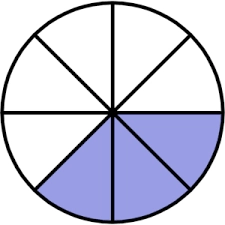# Divide fractions by half

Find the result of division by half:

3/4 : 1/2 =?

x =  3/2 = 1 12

### Step-by-step explanation:Did you find an error or inaccuracy? Feel free to write us. Thank you!

Tips for related online calculators
Need help calculating sum, simplifying, or multiplying fractions? Try our fraction calculator.